# Civil Engineering - Water Supply Engineering

### Exercise :: Water Supply Engineering - Section 5

31.

Alum is a

 A. coagulant B. flocculant C. catalyst D. disinfectant.

Explanation:

No answer description available for this question. Let us discuss.

32.

If L, B and D length, breadth and depth of water in a rectangular sedimentation tank of total discharge Q, the settling velocity, is

 A.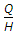B.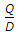C.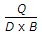D.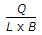E.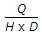Explanation:

No answer description available for this question. Let us discuss.

33.

In a reservoir the average volume of sediment deposition is 0.15 million cubic per year. If the dead storage and total capacity of the reservoir are 8 million cubic metres and 36 million cubic metres respectively,

 A. the reservoir will theoretically be silted in 240 years B. the reservoir will start reducing after 60 years C. both (a) and (b) D. neither (a) nor (b).

Explanation:

No answer description available for this question. Let us discuss.

34.

Q is the discharge from an unconfined tube well with depression head S through a pipe of radius rw. If the radius of influence is R, the minimum required length of the strainer, is

 A.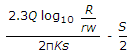B.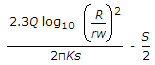C.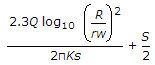D.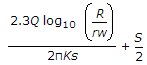Explanation:

No answer description available for this question. Let us discuss.

35.

The requirement of water per capita per day, is

 A. 90 litres B. 150 litres C. 250 litres D. 400 litres.